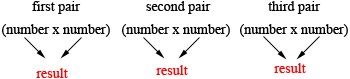SEARCH HOMEMath Central Quandaries & QueriesQuestion from kaylou, a student: Suppose you were given 13 numbers and asked to find their product. Seven of the numbers were positive, and the rest were negative. Would your product be positive or negative?Why?Kaylou,

If 7 are positive then 6 are negative.

When you multiply numbers the order doesn't matter (for example 5 × 7 = 7 × 5) so you can multiply the 7 positive numbers first. Since they are all positive the result will be positive.

Now multiply the 6 negative numbers in pairsAll three results are positive. The final step is to multiply the number which was the product of the 7 positive positive numbers and the three results from the step above.

I hope this helps,
PennyMath Central is supported by the University of Regina and The Pacific Institute for the Mathematical Sciences.